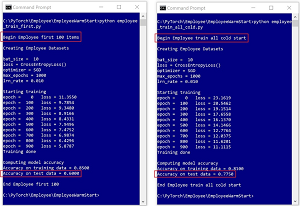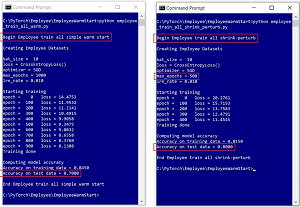Expert Insights for the AI Business Revolution

News

### Warm-Start Training for Machine Learning

The challenge is that when new data arrives periodically, a new prediction model trained using the existing model (a "warm-start"), the resulting new model performs worse than a model trained from scratch (a "cold-start").A research paper titled "On Warm-Starting Neural Network Training" describes a common problem when working with neural network prediction models, and presents a simple but clever technique to overcome that problem. The challenge is that in scenarios where new data arrives periodically, if a new prediction model is trained using the existing model as a starting point (a "warm-start"), which seems like common sense, the resulting new model performs worse than a model trained from scratch (a "cold-start").

The paper was authored by Jordan Ash and Ryan Adams, and was presented at the 2020 Conference on Neural Information Processing (NeurIPS).

The research paper presents a simple solution to the problem. Briefly, when new data arrives to a system, instead of training a new model using warm-start or cold-start, by shrinking the existing model weights towards zero and adding noise, a new model can trained more quickly than cold-starting, and the new model generalizes better than a warm-started model. The research paper calls this technique the shrink and perturb trick. (Research papers often use the word "trick" to indicate a technique, as opposed to a formal algorithm.)

The Problem Scenario
In many machine learning scenarios, new data is continuously arriving into a system and so you need to update your neural network model by retraining it. For example, a system that predicts house prices must be frequently updated to take new sales data into account. Training a neural network is the process of finding the best values of numeric constants, called weights and biases, that define the network.

There are two main retraining options. First, a new model can be trained from scratch using the old existing data combined with the newly arrived data -- cold-start training. The existing neural weights and biases are ignored, and new weights and biases are initialized to small random values. The second option is to train a new model using the combined data, with weights and biases initialized to their existing values -- warm-start training.

You would think that the warm-start training technique would be faster and give a better prediction model than the cold-start training technique. But surprisingly, the warm-start technique consistently works worse -- in the sense that a model that has been trained using warm- start generalizes more poorly than a model trained from scratch. Put another way, when you add new training data, the prediction accuracy of a model trained using warm-start on new, previously unseen data is usually worse than the prediction accuracy of a model trained from scratch.

So, why not just always train from scratch when new data arrives to a system? This is not always feasible. Sophisticated neural prediction systems can have millions, or in the case of natural language processing models, billions of weights and biases. Training such complex models can take weeks or months even on very powerful computing hardware.

It would be nice if there was a training technique that combined the speed of warm-start training with the prediction accuracy of cold-start training. That's exactly what the Warm-Start Training research paper showed how to do.

The Shrink and Perturb Technique
The idea presented in the Warm-Start Training research paper is simple. When new data arrives to a system, the weights and biases of the existing model are initialized by shrinking them towards zero and then adding random noise. To shrink a weight, it is multiplied by a value that's between 0 and 1, typically about 0.5. For example, if one of the weights in the existing model has a value of -1.56 and the shrink factor is 0.5 then the new weight is 0.5 * -1.56 = -0.78.

After shrinking, to perturb a weight or bias, a small random value that is Gaussian distributed with mean 0 and a small standard deviation(such as 0.01) is added. Most perturbation values with a standard deviation of sd will be between (-3 * sd) and (+3 * sd).

A good way to understand the relationship between warm-start, cold-start, and shrink-perturb training is to take a look at concrete examples of cold-start training shown in Figure 1 and warm-start and shrink-perturb training shown in Figure 2.[Click on image for larger view.] Figure 1: (Left) Model trained on initial data has 60% accuracy. (Right) Model trained from scratch with additional data has 77.5% accuracy.

Imagine a problem where the goal is to predict an employee's job ("mgmt," "supp," "tech") based on their sex, age, branch location ("anaheim," "boulder," "concord") and annual income. In Figure 1, a neural network is trained using an initial set of 100 training items. The accuracy of the trained model on a set of held-out test items is 60.00 percent -- not very good as you'd expect for a model trained using only 100 data items.[Click on image for larger view.] Figure 2: (Left) Model trained with additional data using warm-start has only 70% accuracy. (Right) Model trained with additional data using shrink-perturb has 80% accuracy achieved in half the training epochs.

In the right image in Figure 1, 100 additional training items have arrived. A new model is trained from scratch. The accuracy of the new cold-start model on the held-out test data is 77.50 percent -- a significant improvement, as expected because the new model is based on more data.

The left image in Figure 2 shows a new model being trained on the combined 200 data items using warm-start. The accuracy of resulting warm-start model on the held-out test data is only 70.00 percent -- somewhat better than the model trained only on the first 100 data items but surprisingly, worse than the cold-start model.

The right image in Figure 2 shows a new model being trained on the combined 200 data items using the shrink-perturb technique. Even though shrink-perturb training used only 500 epochs (an epoch is one complete pass through the training data) compared to 1,000 epochs on the cold-start and warm-start techniques, the accuracy of the shrink-perturb model on the test data is 80.00 percent -- better than the cold-start technique and obtained using only half the number of training epochs.

Many techniques for neural networks that are developed by research are theoretically interesting but not feasible in practice. But the shrink-perturb technique is simple to implement. For example, for a neural network defined using the PyTorch library, a possible function to apply the shrink-perturb technique is:

```def shrink_perturb(model, lamda=0.5, sigma=0.01):
for (name, param) in model.named_parameters():
if 'weight' in name:   # just weights
nc = param.shape  # cols
nr = param.shape  # rows
for i in range(nr):
for j in range(nc):
param.data[j][i] = \
(lamda * param.data[j][i]) + \
T.normal(0.0, sigma, size=(1,1))
return```

(The code fragment uses spelling "lamda" because "lambda" is a keyword in some programming languages.) With such a function defined, a prediction model can be initialized with the shrink-perturb technique using code like this:

```net = Net().to(device)
fn = ".\\Models\\employee_model_first_100.pth"
shrink_perturb(net, lamda=0.5, sigma=0.01)
# now train net as usual```

The point of these code snippets is to illustrate that the shrink-perturb technique is simple to implement and easy to use in practice.

Wrapping Up
The Pure AI Editors asked Jordan Ash, one of the authors of the Warm-Start research paper, if the work was motivated by theory or the desire to improve machine learning models in practice. He replied, "I'd say a bit of both. The warm-start problem is an important phenomenon, and the shrink-perturb initialization we propose appears to correct it." Ash added, "But our paper only studies these things from an empirical standpoint."

James McCaffrey, from Microsoft Research, reviewed the Warm-Start research paper and commented, "Updating machine learning models based on new data is an important task in production systems. This paper appears to be an important contribution to understanding these scenarios."

Ash commented, "It's possible that there exist initialization schemes that work even better than the shrink-perturb initialization we propose, which is currently much faster than randomly initializing but not quite as fast as pure warm starting."